Next: Angular Momentum Quantum Numbers Up: Spin Previous: The Periodic Table

#### The Zeeman Effect

Place an atom in a magnetic field, and (as Zeeman discovered) its spectral lines will split. Pais's book Inward Bound gives fascinating details of the experimental history.

For some atoms, spectral lines split in three under a magnetic field. This is known as the normal Zeeman effect. For other atoms, the spectrum displays a more complex pattern of splittings, known as the anomalous Zeeman effect. When the magnetic field becomes strong enough, however, some lines merge back together, and the anomalous Zeeman splitting coalesces smoothly into a normal splitting-- this is called the Paschen-Back effect. Young Heisenberg wrestled with the anomalous Zeeman effect, as a student in Sommerfeld's seminar on atomic spectra (see Cassidy's biography Uncertainty.)

The full explanation of all this phenomena presented quite a puzzle to the quantum pioneers. Even today, fitting all the pieces together can be confusing. In the next few sections I will try to assemble this puzzle.

I treat the Zeeman effect in such gory detail for two reasons. First, historical interest: the Zeeman effect gave the first hints of the complexities of quantum spin. Many of the strange spin facts'' I stated earlier were first discovered empirically, from poring over spectra and noticing patterns.

Second, gestalt'': the very complexity of the Zeeman effect means that any explanation of it must bring together several aspects of quantum mechanics.

We can get started by comparing figures 1 and 2. We see that spectral lines will split if energy levels move up or down (i.e., if the energy levels of quantum states change). To progress further, one needs a formula for the energy levels of the states of an atom-- or rather, a formula for the change in energy level due to the magnetic field.

Say we write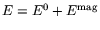, whereis the energy with no magnetic field, and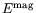is the energy due to the magnetic field. (I will add quantum-number subscripts (like we had with) in a moment.) Here is a formula forthat accounts for the normal Zeeman effect: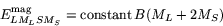(1)

where,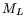,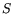, andare quantum numbers that I will discuss below, and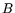is the strength of the magnetic field. Here is a formula that accounts for the anomalous Zeeman effect:(2)

where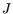and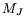are another pair of quantum numbers to be discussed below. The complicated fraction built out of,, andon the right hand side is known as the Landé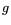-factor.

So there are four pieces to the puzzle:

1. What is the meaning of the six quantum numbers,,,,, and?
2. How do the normal'' and anomalous'' formulas (formulas 1 and 2) account for the details of the Zeeman splitting?
3. Where do the normal and anomalous Zeeman formulas come from?
4. How does the anomalous Zeeman formula turn into the normal Zeeman formula in the Paschen-Back limit of strong magnetic fields?
The next four sections tackle each of these questions in turn.Next: Angular Momentum Quantum Numbers Up: Spin Previous: The Periodic Table